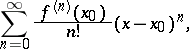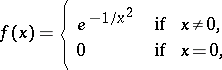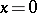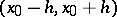# Taylor series

(diff) ← Older revision | Latest revision (diff) | Newer revision → (diff)

A power series(1)

where the numerical functionis defined in some neighbourhood of the pointand has at this point derivatives of all orders. The partial sums of a Taylor series are Taylor polynomials (cf. Taylor polynomial).

Ifis a complex number and the functionis defined in some neighbourhood ofin the field of complex numbers and is differentiable at, then there exists a neighbourhood ofin whichis the sum of its Taylor series (1) (see Power series). If, however,is a real number and the functionis defined in some neighbourhood ofin the field of real numbers and has atderivatives of all orders, then there may be no neighbourhood ofin whichis the sum of its Taylor series. For instance, the function(2)

is differentiable infinitely many times on the entire real axis, is equal to 0 only at the point, but all coefficients of its Taylor series at this point are equal to 0.

If a function is the sum in some neighbourhood of a given point of a power series with centre at that point, then such a series is unique and is the Taylor series of this function at the given point. However, one and the same power series can be the Taylor series of different real functions. Indeed, the power series all coefficients of which are equal to 0 is the Taylor series both of the function identically equal to 0 on the entire real axis and of the function (2) at the point.

A sufficient condition for the convergence of the Taylor series (1) to the real-valued functionon an intervalis the existence of a common bound for all its derivatives in this interval.

The Taylor series can be generalized to the case of mappings of subsets of linear normed spaces into similar spaces, and in particular to numerical functions of several variables and to functions of a matrix argument.

The series (1) was published by B. Taylor in 1715, a series reducible to the series (1) by a simple transformation was published by Johann I. Bernoulli in 1694.

How to Cite This Entry:
Taylor series. Encyclopedia of Mathematics. URL: http://encyclopediaofmath.org/index.php?title=Taylor_series&oldid=15684
This article was adapted from an original article by L.D. Kudryavtsev (originator), which appeared in Encyclopedia of Mathematics - ISBN 1402006098. See original article